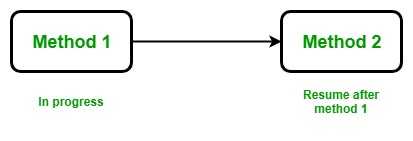• Difficulty Level : Medium
• Last Updated : 22 Apr, 2020

Multitasking is the simultaneous execution of multiple tasks or processes over a certain time interval. Windows operating system is an example of multitasking because it is capable of running more than one process at a time like running Google Chrome, Notepad, VLC player, etc. at the same time. The operating system uses a term known as a process to execute all these applications at the same time. A process is a part of an operating system that is responsible for executing an application. Every program that executes on your system is a process and to run the code inside the application a process uses a term known as a thread.
A thread is a lightweight process, or in other words, a thread is a unit which executes the code under the program. So every program has logic and a thread is responsible for executing this logic. Every program by default carries one thread to executes the logic of the program and the thread is known as the Main Thread, so every program or application is by default single-threaded model. This single-threaded model has a drawback. The single thread runs all the process present in the program in synchronizing manner, means one after another. So, the second process waits until the first process completes its execution, it consumes more time in processing.

For example, we have a class named as Geek and this class contains two different methods, i.e method1, method2. Now the main thread is responsible for executing all these methods, so the main thread executes all these methods one by one.Example:

 `// C# program to illustrate the ``// concept of single threaded model``using` `System;``using` `System.Threading;`` ` `public` `class` `Geek {`` ` `    ``// static method one``    ``public` `static` `void` `method1()``    ``{`` ` `        ``// It prints numbers from 0 to 10``        ``for` `(``int` `I = 0; I <= 10; I++) {`` ` `            ``Console.WriteLine(``"Method1 is : {0}"``, I);`` ` `            ``// When the value of I is equal to ``            ``// 5 then this method sleeps for ``            ``// 6 seconds and after 6 seconds ``            ``// it resumes its working``            ``if` `(I == 5) {``                ``Thread.Sleep(6000);``            ``}``        ``}``    ``}`` ` `    ``// static method two``    ``public` `static` `void` `method2()``    ``{`` ` `        ``// It prints numbers from 0 to 10``        ``for` `(``int` `J = 0; J <= 10; J++) {`` ` `            ``Console.WriteLine(``"Method2 is : {0}"``, J);``        ``}``    ``}``}`` ` `// Driver Class``public` `class` `GFG {`` ` `    ``// Main Method``    ``static` `public` `void` `Main()``    ``{`` ` `        ``// Calling static methods``        ``Geek.method1();``        ``Geek.method2();``    ``}``}`

Output:

```Method1 is : 0
Method1 is : 1
Method1 is : 2
Method1 is : 3
Method1 is : 4
Method1 is : 5
Method1 is : 6
Method1 is : 7
Method1 is : 8
Method1 is : 9
Method1 is : 10
Method2 is : 0
Method2 is : 1
Method2 is : 2
Method2 is : 3
Method2 is : 4
Method2 is : 5
Method2 is : 6
Method2 is : 7
Method2 is : 8
Method2 is : 9
Method2 is : 10
```

Explanation: Here, first of all, method1 executes. In method1 , for loop starts from 0 when the value of i is equal to 5 then the method goes into sleep for 6 seconds and after 6 seconds it resumes its process and print remaining value. Until method2 is in the waiting state. method2 start its working when method1 complete its assigned task. So to overcome the drawback of single threaded model `multithreading `is introduced.Example:

 `// C# program to illustrate the``// concept of multithreading``using` `System;``using` `System.Threading;`` ` `public` `class` `GFG {`` ` `    ``// static method one``    ``public` `static` `void` `method1()``    ``{`` ` `        ``// It prints numbers from 0 to 10``        ``for` `(``int` `I = 0; I <= 10; I++) {``            ``Console.WriteLine(``"Method1 is : {0}"``, I);`` ` `            ``// When the value of I is equal to 5 then``            ``// this method sleeps for 6 seconds``            ``if` `(I == 5) {``                ``Thread.Sleep(6000);``            ``}``        ``}``    ``}`` ` `    ``// static method two``    ``public` `static` `void` `method2()``    ``{``        ``// It prints numbers from 0 to 10``        ``for` `(``int` `J = 0; J <= 10; J++) {``            ``Console.WriteLine(``"Method2 is : {0}"``, J);``        ``}``    ``}`` ` `    ``// Main Method``    ``static` `public` `void` `Main()``    ``{`` ` `        ``// Creating and initializing threads``        ``Thread thr1 = ``new` `Thread(method1);``        ``Thread thr2 = ``new` `Thread(method2);``        ``thr1.Start();``        ``thr2.Start();``    ``}``}`

Output :

```Method1 is : 0
Method1 is : 1
Method1 is : 2
Method1 is : 3
Method2 is : 0
Method2 is : 1
Method2 is : 2
Method2 is : 3
Method2 is : 4
Method2 is : 5
Method2 is : 6
Method2 is : 7
Method2 is : 8
Method2 is : 9
Method2 is : 10
Method1 is : 4
Method1 is : 5
Method1 is : 6
Method1 is : 7
Method1 is : 8
Method1 is : 9
Method1 is : 10
```

Explanation: Here, we create and initialize two threads, i.e thr1 and thr2 using Thread class. Now using `thr1.Start();` and `thr2.Start();` we start the execution of both the threads. Now both thread runs simultaneously and the processing of thr2 does not depend upon the processing of thr1 like in the single threaded model.

Note: Output may vary due to context switching.